Home PHYSICS TOPIC 1: APPLICATION OF VECTORS | PHYSICS FORM 3

# TOPIC 1: APPLICATION OF VECTORS | PHYSICS FORM 3

769
32
SHARE### TOPIC 1: APPLICATION OF VECTORS | PHYSICS FORM 3

Scalar and Vector Quantities

Difference between Scalar and Vector Quantities
Distinguish between scalar and vector quantities
##### Scalar Quantities
These are physical quantities which have magnitude only. Examples of scalar quantities include mass, length, time, area, volume, density, distance, speed, electric current and specific heat capacity.
##### Vector Quantities
These are physical quantities which have both magnitude and direction. Examples of vector quantities include displacement, velocity,
acceleration, force, pressure, retardation, and momentum.
Addition of Vectors Using Graphical Method
Scalar physical quantities have magnitude only. Thus, they can be added, multiplied, divided, or subtracted from each other.
Example 1
If you add a volume of 40cm3 of water to a volume of 60cmof water, then you will get 100cm3 of water.
Vectors can be added, subtracted or multiplied conveniently with the help of a diagram.
Vectors Representation
A vector quantity can be represented on paper by a direct line segment.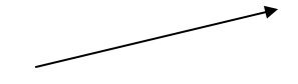1. The length of the line segment represents the magnitude of a vector.
2. The arrow head at the end represents the direction.
There are two methods that are used to sum up two vectors:
1. Triangle method
2. Parallelogram method.
Triangle Method
A step-by-step method for applying the head-to-tail method to determine the sum of two or more vectors is given below.
1.Choose
a scale and indicate it on a sheet of paper. The best choice of scale
is one that will result in a diagram that is as large as possible, yet
fits on the sheet of paper.
2.Pick a starting location and draw the first vectorto scalein the indicated direction. Label the magnitude and direction of the scale on the diagram (e.g., SCALE: 1 cm = 20 m).
3.Starting from where the head of the first vector ends, draw the second vectorto scalein the indicated direction. Label the magnitude and direction of this vector on the diagram.
4.Draw the resultant from the tail of the first vector to the head of the last vector. Label this vector as Resultantor simplyR.
5.
Using a ruler, measure the length of the resultant and determine its
magnitude by converting to real units using the scale (4.4 cm x 20 m/1
cm = 88 m).
6.Measure the direction of the resultant using the counterclockwise convention.
Resultant vector: This
is the vector drawn from the starting point of the first vector to the
end point of the second vector which is the sum of two vectors.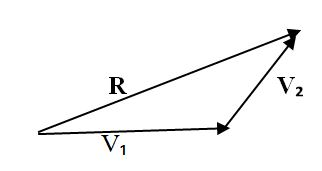Where:
• Vi – First vector
• V2 – Second vector
• R – Resultant vector
Example 2
Suppose a man walks starting from point A, a distance of 20m due North, and then 15m due East. Find his new position from A.
Solution
Use scale
1CM Represents 5m
Thus
20m due to North Indicates 4 cm
15m due to East Indicates 3cm.
Demonstration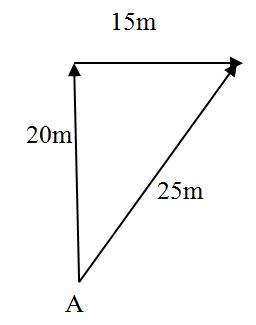The position of D is represented by Vector AD of magnitude 25M or 5CM at angle of 36051”
Since
• Tan Q = (Opposite /Adjacent)
• Tan Q = 3cm /4cm
• Q = Tan -1 (3/4)
• Q = Tan -1(0.75)
• Q = 35º51”
The Resultant displacement is 25m ad direction Q = 36º51”
The Triangle and Parallelogram Laws of Forces
State the triangle and parallelogram laws of forces
Triangle Law of Forces
Triangle
Law of Forces states that “If three forces are in equilibrium and two
of the forces are represented in magnitude and direction by two sides of
a triangle, then the third side of the triangle represents the third
force called resultant force.”
Example 3
A
block is pulled by a force of 4 N acting North wards and another force
3N acting North-East. Find resultant of these two forces.
Demonstration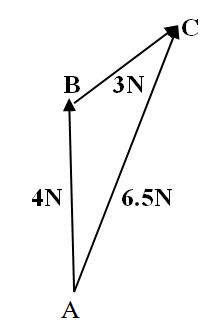Scale
1Cm Represents 1N
Draw a line AB of 4cm to the North. Then, starting from B, the top vectorofAB, draw a line BC of 3 CM at 45oEast of North.
Join
the line AC and measure the length (AC = 6.5 cm) which represents 6.5N.
Hence, AC is the Resultant force of two forces 3N and 4N.
Parallelogram Method
In
this method, the two Vectors are drawn (usually to scale) with a common
starting point , If the lines representing the two vectors are made to
be sides of s parallelogram, then the sum of the two vectors will be the
diagonal of the parallelogram starting from the common point.
The
Parallelogram Law states that “If two vectors are represented by the
two sides given and the inclined angle between them, then the resultant
of the two vectors will be represented by the diagonal from their common
point of parallelogram formed by the two vectors”.
Example 4
Two
forces AB and AD of magnitude 40N and 60N respectively, are pulling a
body on a horizontal table. If the two forces make an angle of 30o between them find the resultant force on the body.
Solutiuon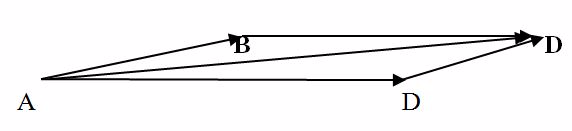Choose a scale.
1cm represents10N
Draw a line AB of 4cm
Draw a line AD of 6cm.
Make an angle of 30between AB and AD. Complete the parallelogram ABCD using the two sides AB and include angle 30O.
Draw the lineAC with a length of9.7 cm, which is equivalent to 97 N.
The lineAC of the parallelogram ABCD represents the resultant force of AB and AD in magnitude and direction.
Example 5
Two
ropes, one 3m long and the other and 6m long, are tied to the ceiling
and their free ends are pulled by a force of 100N. Find the tension in
each rope if they make an angle of 30o between them.
Solution
1cm represents 1N
Thus
3cm = represent 3m
6cm = represents 6m
DemonstrationBy using parallelogram method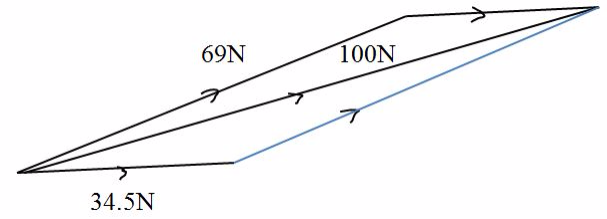Tension, determined by parallelogram method, the length of diagonal using scale is 8.7 cm, which represents 100N force.
Thus.
Tension in 3m rope = 3 X 100 / 8.7 = 34.5N
Tension in 6m rope = 6 x 100 / 8.7 =69N
Tension force in 3m rope is 34.5N and in 6m rope is 69N.
NoteEquilibrant forcesare those that act on a body at rest and counteract the force pushing or pulling the body in the opposite direction.

1. Hey I know this is off topic but I was wondering if you knew of any widgets I could add to my blog that automatically tweet my newest twitter updates.
I’ve been looking for a plug-in like this for
quite some time and was hoping maybe you would have some
experience with something like this. Please let me know if you run into anything.

2. Hello, for all time i used to check website posts here in the early hours in the dawn, as i like to learn more and more.

3. I love what you guys are usually up too. This sort of clever work and exposure!
Keep up the terrific works guys I’ve incorporated you guys to my personal blogroll.

4. We are a group of volunteers and starting a new scheme in our community.
Your site provided us with valuable info to work on. You have done an impressive job and our whole community will be thankful to you.

5. I got this website from my friend who told me regarding this site and now this time I
am browsing this web page and reading very informative articles here.

6. Hello, i read your blog occasionally and i own a similar one
and i was just curious if you get a lot of spam comments?

If so how do you reduce it, any plugin or anything you can suggest?

I get so much lately it’s driving me insane so any assistance is very much appreciated.

7. It’s difficult to find experienced people in this particular subject,
however, you sound like you know what you’re talking about!
Thanks